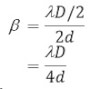# Short Questions

1. Explain why two flash light held closed together will not produce an interference pattern on a distance screen?
An interference pattern is obtained only when the two flash lights is coherent is Two sources must emit continuous light waves of same frequency, wave length, amplitude and have zero phase. But it is not possible for two independent source to be coherent. Hence they will not produce an interference pattern.

2. Can two independent sources of light produce interference of light?
No, two independent sources of light cannot produce interference of light because to produce interference of light two sources must be coherent i.e. having same frequency wave length and of some phase but two independent source of light cannot be coherent. Hence they cannot produce interference of light.

3. Why have two sources of light to be close to each other for the production of good interference?
We know that,
b = λD/d, i.e.is inversely proportional to the separation of two sources of light. So, if the sources are close to each other b increases and fringes appears with grate width which is good to see. Hence the interference is good.

4. If young’s apparatus is immersed in water, what will be the effect of fringe width?
If young’s apparatus is immersed in water, the wavelength of light decreases. We know that, b = λD/d i.e. b is directly proportional to l. Hence if young’s apparatus is immersed in water, fringe width will decrease due to decrease in wavelength of light.

5. In young’s spit experiment, the separation of slit is doubled and distance between the slit and screen is halved. How will be the effect of fringe width?
We have, b = λD/d, if the separation of slit is doubled and distance between the slit and screen is halvedHence the fringe width will be one fourth.